Derivation of Page 3 Equations

Consider an object launched with some velocity Vo at an angle q measured above the horizontal.  We can  resolve the vector into orthogonal (means mutually perpendicular) components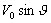vertically and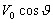horizontally.
Because orthogonal vectors add to form a sum but do nothing to increase one another, we can apply our trusty page 1 equations to get some useful relationships.

From page 1, line 1 we know that V = Vo + at
This equation reduces to two other equations (1)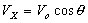and (2)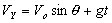From page 1, line 3 we know that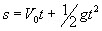By substituting the horizontal and vertical components of the velocity of projection we get
(3)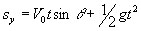and  (4)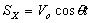Finally, if we solve equation (4) for t and substitute this fraction for t in equation (3), we get

(5)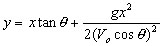qwertyuioopasdfghjklzxcvbnmQWERTYUIOPASDFGHJKLZXCVBNM